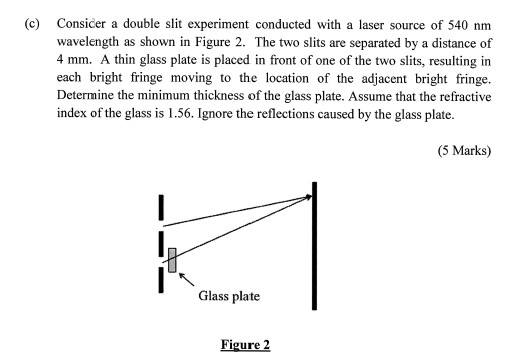# Double Slit Question with Thin Lens concept

## Homework Statement

Consider a double slit experiment with a light source of 540nm. Slit separation is 4mm. A thin glass plate is placed in front of one of the slit,, resulting in each bright fringe moving to the location of the adjacent bright fringe. Find the minimum thickness of the glass.

Refractive index of the glass is 1.56. Ignore reflections caused by the glass.

## Homework Equations

2nt= +- mλ (bright fringe)
2nt= +- m+½λ (dark fringe)

## The Attempt at a Solution

I think I should be using the thin lens formula since they emphasised the thin glass plate as "thin". Bright fringe moves the distance of a dark fringe to take the position of the adjacent bright fringe.

It seems too simple to just use

t= (m+½λ)/2n

Is there something I am missing?

BvU
Homework Helper
Hello John,

No lens. But I don't see what minimum thickness you propose. m, t, n ?

By the way, I'd like to see a drawing of the situation before and after placing the glass plate. I have difficulty understanding what exactly happens with the 1st maximum (does it move to where the 2nd was before?) Then how can the 2nd maximum move to where the 3rd was instead of to where the 4th was ?

Hello John,

No lens. But I don't see what minimum thickness you propose. m, t, n ?

By the way, I'd like to see a drawing of the situation before and after placing the glass plate. I have difficulty understanding what exactly happens with the 1st maximum (does it move to where the 2nd was before?) Then how can the 2nd maximum move to where the 3rd was instead of to where the 4th was ?

It's a conceptual question though.I merely used:

Thickness= λ/4n

to obtain 8.65×10^-8 m

BvU